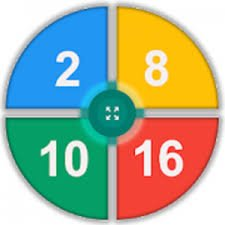# Number System for Students v1.9 [BCA,MCA,B.Tech,M.Tech]Sharing is Caring ッ
•
•
•
•
•
•

## Number System for Students

Number System for Students is the best app for engineering, computer science and IT guys like BCA, MCA, B.Tech, M.Tech college students. In this app, you can easily convert an integer or fractional number into the binary, decimal, octal or hexadecimal system. It’s not only an educative tool, it’s also a calculator and conversion tool.

### WORK LIST OF NUMBER SYSTEM FOR STUDENTS APP:

★ Convert binary (Integer or fractional) number into the decimal, octal or hexadecimal system.
★ Convert decimal (Integer or fractional) number into the binary, octal or hexadecimal system.
★ Convert octal (Integer or fractional) number into the binary, decimal or hexadecimal system.
★ Convert hexadecimal (Integer or fractional) number into the binary, decimal or octal system.
★ Multiple language support (English or Bangla).
★ Simple UI.
★ Solution for each result.

#### What’s new:

• Minor Bug fixes